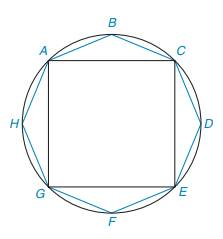Chapter 8.3, Problem 34E### Elementary Geometry for College St...

6th Edition
Daniel C. Alexander + 1 other
ISBN: 9781285195698

#### Solutions

Chapter
Section### Elementary Geometry for College St...

6th Edition
Daniel C. Alexander + 1 other
ISBN: 9781285195698
Textbook Problem
37 views

# Regular octagon ABCDEFGH is inscribed in a circle whose radius is 7 2 2 cm. Considering that the area of the octagon is less than the area of the circle and greater than the area of the square ACEG, find the two integers between which the area of the octagon must lie.(Note: For the circle, use A = π r 2 with π ≈ 22 7 .)To determine

To find:

The two integers between which the area of the octagon must lie.

Explanation

1) The diameter of the circle is twice the radius.

2) The area of the circle is given by, A=πr2 where r is the radius of the circle.

3) The area of the square is given by, A=d22 where d is diagonal of the square.

Calculation:

It is given that the radius of the circle is 722cm.

Use the formula to find the area of the circle, A=πr2

Substitute r=722 in A=πr2

Acircle=π(722)2=49π2=492×227=77cm2

The diameter of the circle is given by 72cm

### Still sussing out bartleby?

Check out a sample textbook solution.

See a sample solution

#### The Solution to Your Study Problems

Bartleby provides explanations to thousands of textbook problems written by our experts, many with advanced degrees!

Get Started

#### Find more solutions based on key concepts